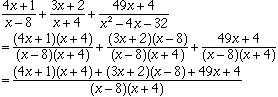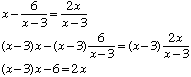Name: leslie Who is asking: Student Level: Secondary Question: ```add 4x+1 3x+2 49x+4 over + over + over x-8 x+4 x^2-4x-32 6 2x solve x- over = over x-3 x-3 ``` Hi Leslie, Working with algebraic fractions is very similar to working woth arithmetic fractions. To add fractions they need the same denominator. You have denominators x-8, x+4 and x2-4x+32. But x2-4x+32 = (x-8)(x+4) so if you multiply the numerator and denomonator of the first fraction by x+4 and the numerator and denomonator of the second fraction by x-8 then all three fractions will have the same denominator. That isNow expand the numerator and simplify. For your second problem I would multiply both sides by x-3 and then solve. ThusSolve this equation for x and then verify by substituting into the original equation. Cheers, Penny Go to Math Central